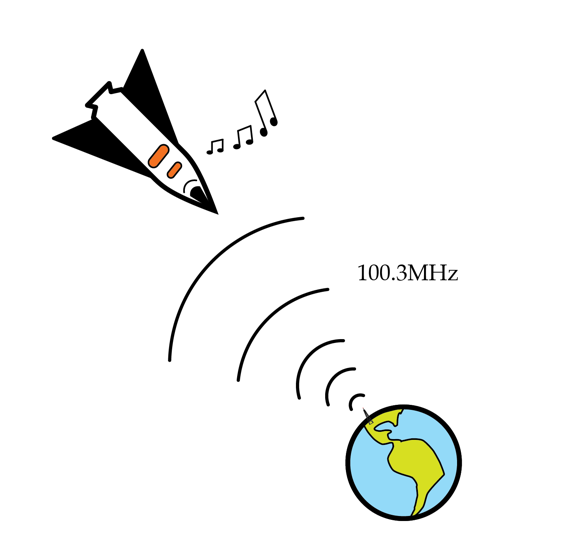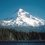# Space Oddity RatioDao Z. submitted such an excellent solution to last week's Problem of the Week that we decided to feature it as the solution to our problem of the week. We were impressed by the amount of thought he put into stating it clearly and completely.

If you solved last week's Problem of the Week correctly, you were entered into a raffle for a free t-shirt. We will keep you posted as winners are announced. Feel free to discuss either the question or the solution here.

To recap, David told us about the sound quality of his favorite radio station during his last vacation in the following problem:

I'm in a spaceship very far away from earth but traveling straight towards earth with a speed v. It's boring out here, so I decide to try and tune in to some of my favorite earthly radio stations. I remember that my favorite station has a frequency of 100.3 MHz and so tune my radio to exactly this frequency. Amazingly, I hear the radio station just like I do on earth! How fast is my spaceship going in m/s? (Hint: it's not that fast...I think I should check whether my engines are on).

### Assumptions

1. Photons of electromagnetic radiation have an intrinsic kinetic energy related to their frequency by $E=hf$ where $h$ is Planck's constant.
2. The gravitational interaction between photons and earth can be treated via usual Newtonian gravity and $E=mc^2$ to convert between energy and mass.
3. The total energy of the photons is conserved.
4. The earth can be treated as a sphere of radius 6370 km and mass $6 \times 10^{24}~kg$. You can ignore rotation of the earth.
5. The speed of light is $3 \times 10^8~m/s$.

Space would probably be a much lonelier place without earth radio.

Here is Dao Z.'s solution (only minor paragraphing edits were made):

### Dao Z.'s Solution:

I will explain what is going on in the question. Radio signal of frequency $f_0$ is emitted from the Earth. One photon in such a signal thus has the intrinsic energy (excluding the potential energy) of $hf_0$. Since the spaceship is "very far away", I assume that the photon travels a distance long enough to break free from the gravitation of the Earth. Hence the poor photon spent some of its intrinsic energy overcoming the Earth's gravitational pull, i.e. gravitational potential energy, and hence the frequency drops to $f'$.However, the spaceship is traveling towards the source of the signal, so according to the doppler effect, the apparent frequency to the people in the spaceship should be higher than the actual frequency of the signal reaching them. Therefore, $f'$ increases to $f_0$ again by doppler effect when the spaceship is travelling at a speed of $v$. Now what we are going to do is to find $v$. Initial energy of the photon on Earth=intrinsic energy + gravitational potential energy. i.e. Initial energy $=hf_0 + (-\frac{GMm}{R})$ , where $M$ is the mass of the Earth, and $m=\frac{hf_0}{c^2}$ is the "mass" of the photon according to $E=mc^2$, and $R$ is the radius of the Earth.

When the signal reaches the spaceship, the gravitational potential energy of the photon becomes 0 because we assume that the photon is now at a "infinite" distance away from Earth and free of gravitational interaction with any other objects. The photon now has the new frequency, $f'$ and the new energy $hf'$. By conservation of energy, $hf'=hf_0 + (-\frac{GMm}{R})$. Sub $m=\frac{hf_0}{c^2}$ to get:

$hf'=hf_0 - \frac{GMhf_0}{c^2 R}$

$f'=f_0 - \frac{GMf_0}{c^2 R}=(1-\frac{GM}{Rc^2})f_0$

$\frac{f'}{f_0}=1-\frac{GM}{Rc^2}$

According to the formula of the doppler effect, $f_0=\frac{c+v}{c}f'$,$\frac{f'}{f_0}=\frac{c}{c+v}=1-\frac{v}{c+v}$. Since c>>v, $c+v \approx c$, and sub $\frac{f'}{f_0}=1-\frac{GM}{Rc^2}$ back to get: $1-\frac{v}{c}=1-\frac{GM}{Rc^2}$ and thus $v=\frac{GM}{Rc}$. When we sub all the values in, we get $v\approx 0.209$, which is the answer.

Appendix: Actually when dealing with light, we have to use relativistic doppler effect, because speed of light is the same in any inertial frame, but since v is so small, the relativistic doppler effect can be simplify to the normal doppler effect. Here is the proof: According to the formula of the relativistic doppler effect, the apparent frequency, $f_0=\sqrt{\frac{c+v}{c-v}}f'$ and $\frac{f'}{f_0}=\sqrt{\frac{c-v}{c+v}}=\sqrt{1-\frac{2v}{c+v}}$ , where c is the speed of light and v is speed of the spaceship towards the Earth. Since c>>v, $\frac{2v}{c+v}$ is very small, so by the binomial expansion to the first two term, $f_0=\sqrt{1-\frac{2v}{c+v}}f' \approx (1-(\frac{1}{2})(\frac{2v}{c+v}))f'=(1- \frac{v}{c+v})f'=\frac{c}{c+v}f'$, in accordance to the normal doppler effect equation. Hence the result will be the same.

We thought that was a nice explanation.Note by Peter Taylor
6 years, 6 months ago

This discussion board is a place to discuss our Daily Challenges and the math and science related to those challenges. Explanations are more than just a solution — they should explain the steps and thinking strategies that you used to obtain the solution. Comments should further the discussion of math and science.

When posting on Brilliant:

• Use the emojis to react to an explanation, whether you're congratulating a job well done , or just really confused .
• Ask specific questions about the challenge or the steps in somebody's explanation. Well-posed questions can add a lot to the discussion, but posting "I don't understand!" doesn't help anyone.
• Try to contribute something new to the discussion, whether it is an extension, generalization or other idea related to the challenge.

MarkdownAppears as
*italics* or _italics_ italics
**bold** or __bold__ bold
- bulleted- list
• bulleted
• list
1. numbered2. list
1. numbered
2. list
Note: you must add a full line of space before and after lists for them to show up correctly
paragraph 1paragraph 2

paragraph 1

paragraph 2

[example link](https://brilliant.org)example link
> This is a quote
This is a quote
    # I indented these lines
# 4 spaces, and now they show
# up as a code block.

print "hello world"
# I indented these lines
# 4 spaces, and now they show
# up as a code block.

print "hello world"
MathAppears as
Remember to wrap math in $$ ... $$ or $ ... $ to ensure proper formatting.
2 \times 3 $2 \times 3$
2^{34} $2^{34}$
a_{i-1} $a_{i-1}$
\frac{2}{3} $\frac{2}{3}$
\sqrt{2} $\sqrt{2}$
\sum_{i=1}^3 $\sum_{i=1}^3$
\sin \theta $\sin \theta$
\boxed{123} $\boxed{123}$